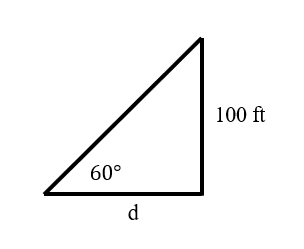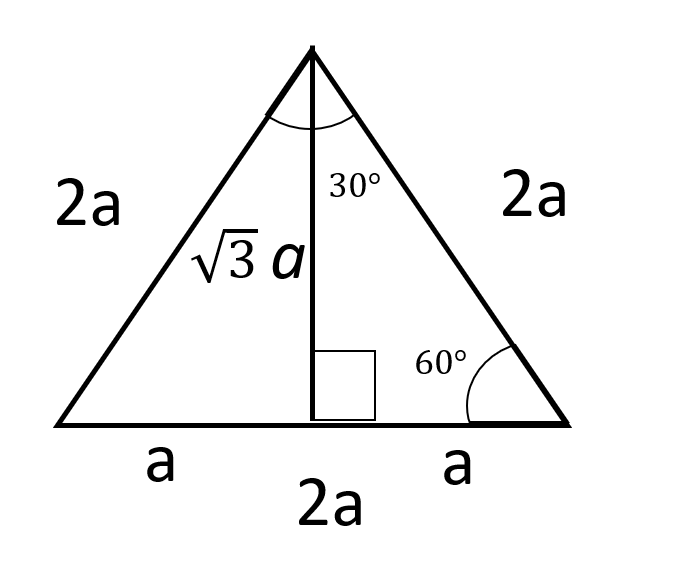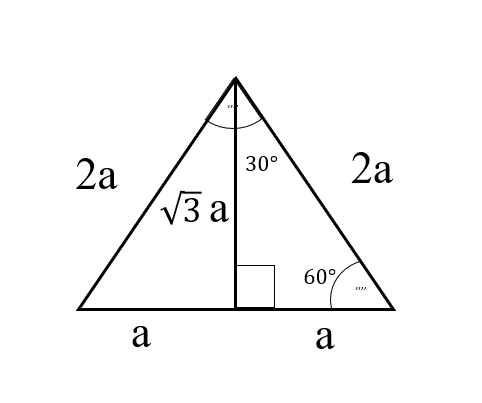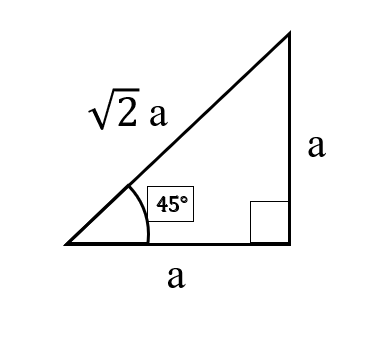Trigonometry help | Trigonometry Homework Help | Trigonometry Problem Solvers

## Top Questions

t schedule 3 people for the day my % scheduled is 78.57% My question is how did the computer come up with the 78.57%?
View More

I would love to get a written solution. I did the other proof tree that you can see in the attaached files. So its supposed to look like that Thank You.
View More

xtbook. i really need in in half an hour
View More

## Core Trigonometric Lessons

### Trigonometric Identities: -

Trigonometric identities are the basic relation between the trigonometric ratio sinθ,cos , tanθ, cotθ, secθ, and cosecθ. Which are  θ+ =1

1+ =

1+ =cosec2

### Double angle formula: -

These formulae are used to solve trigonometric functions and equation.
Sometimes used to calculate the value for trigonometric value of higher angle.
sin2A=2sinAcosA
cos2A=A -A
tan 2A =2tan A 1-A

### Half angle formula: -

These formulae are used to solve trigonometric functions and equation.
Sometimes used to calculate the value for trigonometric value of smaller angle.
sinA=2sin A2 cos A2
cosA=A2 -A2

### Trigonometry function: -

Trigonometric function relates sides of triangle with the angle of that triangle, they often called as circular function also. The six trigonometry functions are sine, cosine, tangent, cotangent, secant, and cosecant.

### Pythagoras Theorem: -

This theorem relates three sides of the right angled triangle, which sates that square of the longest side of the triangle is equal to the sum of the square of the other two sides.

### Right triangle: -

This a type of a triangle on the basis of angle and in this one we say, if one angle of triangle is 90° then that triangle is known as right angled triangle.

### Trigonometric equation: -

Equations in which we have trigonometric functions are known as trigonometric equation. In order to solve them we use trigonometric identities, formulae and some concept of inverse trigonometry.

### Unit circle introduction: -

Unit circle is circle with radius one and used in trigonometry to find the values and signs of higher angle. Using it we can also derive formulae of trigonometry.

### Non right triangles: -

All other types of triangles which are not right triangle can be called as no right triangles it includes all acute angled triangle and obtuse angled triangle.

## Sample Trigonometry Problems

Question 1 - A Tower is 100 ft high. At a certain distance a person is standing and notices that the angle of elevation with the ground to the top of the Tower is 60°. How far is the person from the base of the Tower?

Solution 1 - Given,We have height of the Tower = 100 ft

The angle of elevation to top of the Tower = 60°

Let the distance of person form base of Tower is ‘d’

Now,

Tangent 60° = 100/d

√3 = 100d    => d  = 100/√3   => d  = 1001.732 => d  = 57.73672

Question 2 - From the top of a tall building of height 40 m, the angle of depression of the top of small tower is 50° whose height is 15 m. Find the distance between the two buildings.

Solution 2 - Given,

Height of the building = 40 m

Height of the tower = 15 m

Angle of  depression  = 50°

Let the distance between tower and the building is ‘d’

Now,

Tangent 50° = 30/d

1.1917 = 30/d => d  = 30/1.1917=> d  = 25.1741

## Study Applications of Trigonometry at TutorEye

Trigonometry is derived from the Greek words -

TRI       +      GON      +      METRON

(three)         (sides)           (measures)

Trigonometry means measures of three sides and their relationship with angles. It is mostly used in right angled triangles.

There are many applications of trigonometry in real life. In all the situations, distances or heights can be found by using some mathematical techniques which come under the branch of mathematics called Trigonometry. Trigonometry is used to measure:

• the height of a building or mountains.
• used in construction.
• in navigation in the sea.
• used is physics and engineering.
• used in marine biology, criminology.
• solving complex equations & integrals of mathematics

Trigonometric Ratios:

Sine = Opposite/Hypotenuse                  Cosecant = Hypotenuse/OppositeTrigonometric ratios for an angle:

Sine 60° = √3a/2a = √3/2                               Secant 60° = 2/1

Cosecant 60° = 2/√3                                      Tangent 60° = √3a/a = √3/1

Cosine 60° = a/2a = 1/2                                 Cotangent 60° = 1/√3Trigonometric ratios for an angle:

Sine 30° = a/2a = 1/2                                   Cosecant 30° = 2/1

Cosine 30° = √3a/2a = √3/2                         Secant 30° = 2/√3

Tangent 30° = a/√3a = 1/√3                          Cotangent 30° = 1/√3Trigonometric ratios for an angle:

Sine 45° = a/√2a = 1/√2                                       Cosecant 45° = √2a/a = √2 1

Cosine 45° = a/√2a = 1/√2                                   Secant 45° = √2a/a = √2 1

Tangent 45° = aa = 11                                          Cotangent 45° = a/a = 1/1If you are a parent wanting to help your kid with trigonometry homework or if you are a high school student struggling with High School Trigonometry, don’t hesitate to seek assistance and guidance from our Trigonometry problem solvers.

By advancing knowledge of Trigonometry students can open several career options for them at a later stage such architecture, radiology, and navigation.

Sometimes due to lack of guidance provided to students and in other cases due to high reliability on free online Trigonometry help, students may end up finding Trigonometry to be a hard topic.

Sometimes the method of teaching followed in school is not personalized to match your child’s learning style and, in the end, students find themselves struggling to comprehend Trigonometry. This is the starting point of fear as your child does not get the concepts, methods and techniques properly and hence may not be able to attempt trigonometry questions and problems and may lose confidence overall in the subject.

But we at TutorEye pay special attention to our teaching style. Our trigonometry tutors teach students with such methods which are easy to grasp and memorize. Our written help service provides detailed step by step breakdown so that students gain insight on problems and improve their confidence at the same time.

If you are wondering about applications of trigonometric graphs, then take help from our tutors right away. Go over your homework questions and chat with our tutors to seek written solutions to help you map out your problems.

## How to Learn Trigonometry in Few Simple Steps:

To get help from our trigonometry homework solvers, follow the below process:

Step 1: Upload your homework questions and list out the topics you need help with on the form

Step 2: Hire a tutor who is willing to meet your submission deadline and also reach a mutual agreement on price

Step 3: Duly consider the assignment handed over to you before releasing payment from escrow

We do not provide plagiarized material, so our work is 100% original and of the highest quality. To avoid any disputes, we follow a Money Refund policy. Select the best helpers now!

### How do you find the missing side using trigonometry?

We use trigonometric ratio in order to find the missing side of the triangle if we have one side say adjacent then by using tangent ratio we can find the opposite side and by using cosine ratio we can find the hypotenuse.

### How do you calculate trigonometric ratios?

Trigonometric ratio is the ratio of the sides of the right-angled triangle we named the three sides as the opposite, adjacent, and the hypotenuse, a ratio of these three sides with each other are known as a trigonometric ratio.

### What is the easiest way to learn trigonometry?

In order to learn trigonometry, first we should learn about the ratios how to find them if we have sides and If we have an angle, then the relationship between the two.

### How to learn trigonometry?

Learning Trigonometry is easy, all you need is the right approach. After all Trigonometry is all about the relationship between sides and angles of the triangles. Here’s what you need to do:

• Start from scratch by reviewing basic arithmetic, algebra and geometry
• Understand the role of Sine, cosine and tangent functions
• Learn the trigonometry table
• Solve textbook questions
• Focus on the solved examples

You can also learn with our reputed academicians at TutorEye by getting step-by-step explanations on difficult topics. This way learning trigonometry becomes easy and fun!

### How hard is trigonometry?

Just like any other branch of Math, Trigonometry is hard only if you don't practice or have a weak foundation or need help in the basics of the subject.

So to conquer a subject like Trigonometry, you must know:

• Pythagoras theorem
• Be well versed with right angled triangle problems
• Know the basic functions and trigonometry table
• Invest time in solving old test papers
• Practicing equations daily

To reduce the difficulty, you can also get regular Trigonometry homework help from our trusted professionals. Remember the subject is only tough if you consider it to be so. Let’s get into the habit of doing each question and see how you flourish under our expert guidance.

### Who invented trigonometry?

According to the web, it was Hipparchus of Nicaea who is regarded as the “father of Trigonometry”. He even tabulated the values of the corresponding arc and chord for a series of angles. It is also said that the driving force behind inventing Trigonometry was Astronomy. Menelaus and Ptolemy are also known as important contributors in the field of Trigonometry.

### What is trigonometry used for?

Trigonometry is used for solving measurement problems. It is also used in:

• Marine Engineering
• To study Physics, Calculus
• Criminology
• Marine Biology
• Satellite systems
• Cartography
• Oceanography

Infact, if you want to measure a mountain or the height of the building, trigonometry applications will come into play. So take help in Trigonometry from qualified helpers to gain mastery in time.

### When do you learn trigonometry?

Well as a student you are laying foundation to learn Trigonometry as you perform basic mathematical operations in arithmetic, algebra and geometry. As a subject it is introduced  in higher grades.

Along with our competent solvers, you can take help in this subject back and forth, whenever you want. Remember, at TutorEye, you can learn Trigonometry anytime, anywhere.